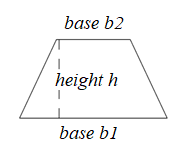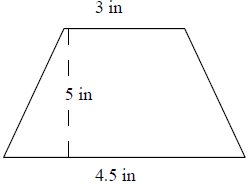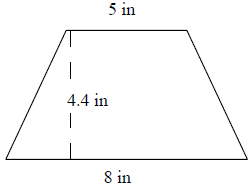# Area of a trapezoidThe trapezoid has one pair of parallel opposite sides called the bases b1 and b2. The height h of the trapezoid is perpendicular distance between these bases.

Formula to find area of a trapezoid

Area of a trapezoid = $\left [ \frac{\left ( b_1 + b_2\right )}{2} \right ] \times h$

where h is the height and b1 and b2 are the bases.

We multiply the average of the bases of the trapezoid with the height of the trapezoid to get its area.

Find the area of the following trapezoid.### Solution

Step 1:

Area of Trapezoid = $\frac{1}{2}$ × h × (b1 + b2); b1, b2 = bases = 3, 4.5; h = height = 5.

Step 2:

Area of trapezoid = $\frac{1}{2}$ × 5 × (3 + 4.5) = 18.75 square in

Find the area of the following trapezoid.### Solution

Step 1:

Area of Trapezoid = $\frac{1}{2}$ × h × (b1 + b2); b1, b2 = bases = 5, 8; h = height = 4.4.

Step 2:

Area of trapezoid = $\frac{1}{2}$ × 4.4 × (5 + 8) = 28.6 square in